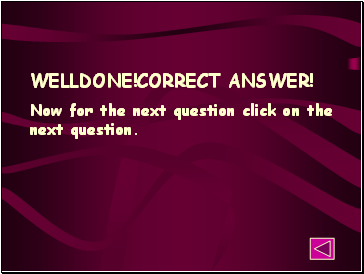# Geometrical shapesPage 2

#### WATCH ALL SLIDES

O

OA = OB = OC = OD

Slide 12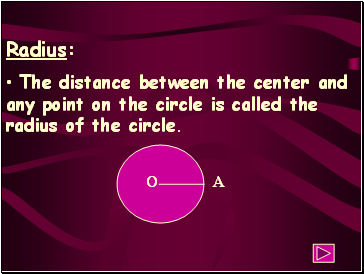The distance between the center and any point on the circle is called the radius of the circle.

AAAA

A

A

O

Slide 13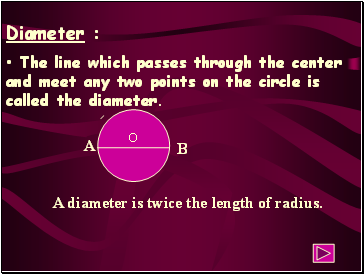## Diameter

The line which passes through the center and meet any two points on the circle is called the diameter.

A

B

A diameter is twice the length of radius.

O

Slide 14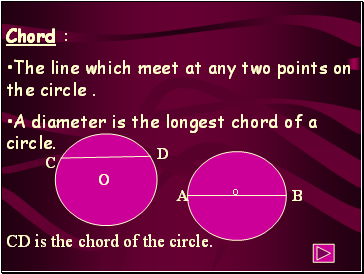## Chord

The line which meet at any two points on the circle .

A diameter is the longest chord of a circle.

CD is the chord of the circle.

A

B

C

D

O

o

Slide 15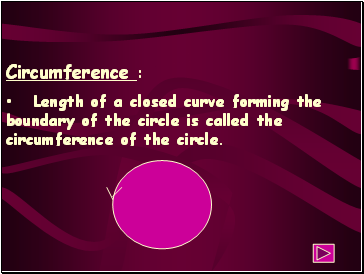## Circumference

Length of a closed curve forming the boundary of the circle is called the circumference of the circle.

Slide 16Slide 17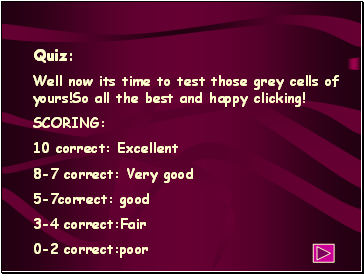Quiz:

Well now its time to test those grey cells of yours!So all the best and happy clicking!

SCORING:

10 correct: Excellent

8-7 correct: Very good

5-7correct: good

3-4 correct:Fair

0-2 correct:poor

Slide 18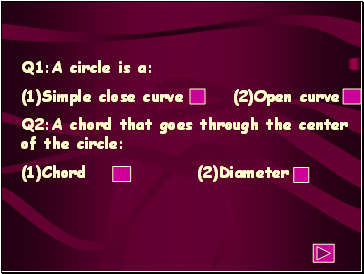Q1:A circle is a:

(1)Simple close curve (2)Open curve

Q2:A chord that goes through the center of the circle:

(1)Chord (2)Diameter

Slide 19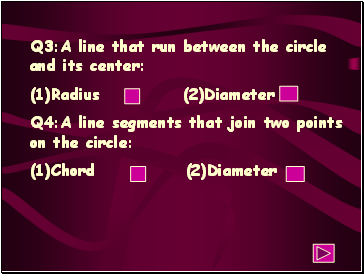Q3:A line that run between the circle and its center:

Q4:A line segments that join two points on the circle:

(1)Chord (2)Diameter

Slide 20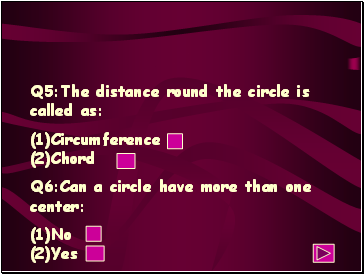Q5:The distance round the circle is called as:

(1)Circumference (2)Chord

Q6:Can a circle have more than one center:

(1)No (2)Yes

Slide 21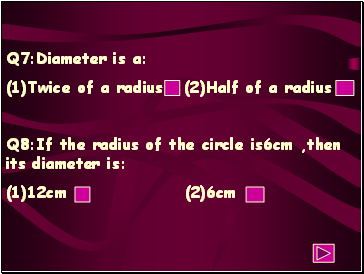Q7:Diameter is a:

Q8:If the radius of the circle is6cm ,then its diameter is:

(1)12cm (2)6cm

Slide 22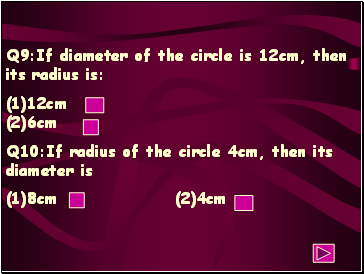Q9:If diameter of the circle is 12cm, then its radius is:

(1)12cm (2)6cm

Q10:If radius of the circle 4cm, then its diameter is

(1)8cm (2)4cm

Slide 23